## Thursday, 29 September 2011

### Chemistry note: Kinetics III - catalyst and gas volume calculations

To access the document version of this chapter of notes click the "Notes Corner" above.

Catalyst effect on rate of reaction
Catalyst is defined as the substance that alters the rate of reaction. Positive catalyst increases while negative catalyst decreases the rate of reaction. In general A+BC is completed with catalyst by A+catalystA-catalyst and react with B to form C. The activation of the reaction path is lower so that higher portion of molecules has enough energy for reaction to occur, hence faster rate of reaction. Feature of catalyst:
-          Not used up in reaction (always regenerated)
-          Only need a small amount in the reaction mixture
-          Does not affect yield but it only affect rate only
-          Often specified for a certain reaction only
-          Increasing surface area for solid catalyst raises the catalytic effect
-          Maybe “poisoned” by small amount of other impurities with decreasing catalytic effect.
Heterogeneous catalyst: catalyst is in different phase with the reactants (i.e. not miscible in simple meaning, though not exactly), e.g. iron for Haber process and V2O5 for contact process.
Principle of heterogeneous catalyst: Catalyst adsorb reactants and weaken their bond and producing more reactive fragment; and the concentration of reactant around the catalyst increase, which increase the effective collision frequency and increase the rate.
Homogeneous catalyst: the catalyst is in the same phase with the reactant, like hydrogen ion in esterfication. The principle is that the catalyst takes part in the reaction mechanism which changes the rate significantly. For example the redox reaction 2I- + S2O82- I2 + 2SO42-, the rate of reaction is slow due to electrostatic repulsion, but under the catalyst iron(II) or (III), the reaction speeds up as iron ion breaks the reaction into two in which positively and negatively ion attracts by the following reaction:
1)       S2O82- + 2Fe2+ 2SO42- + 2Fe3+
2)       2I- + 2Fe3+ I2 + 2Fe2+ (Note: iron(II) is regenerated)
Catalytic convertor is frequently used to reduce pollutants like rhodium and platinum in car catalytic convertor, changing NO, CO and unconsumed hydrocarbon into CO2 and H2O by 2NO+2CON2+2CO2.
Enzyme is a type of biological catalyst and one of the typical enzyme is the yeast decomposing maltose and glucose to give ethanol and carbon dioxide by:
1)       (maltose)C12H22O11 + H2O 2C6H12O6 (glucose)
2)       C6H12O6 2EtOH (ethanol) + CO2
It’s more environmental-friendly like lower temperature required (they usually function in environment slightly hotter than r.t.) and less metal consumed. Some of them are stereo-specific as well in body mechanisms.
Gas volume calculations
Avogadro’s law states that all gases, under same temperature and pressure, contain equal moles of gases (and equal number of particles).
Molar volume is the volume occupied by 1 mole of gas. We can determine by the following experiment: React Mg and HCl of known mass in a closed flask connected to a syringe. Measure volume and number of moles of H2 released, the syringe conserves the pressure inside and outside the flask so the volume-mole ratio is recorded under atm.
In 298K and 1atm, the molar volume of [ideal] gas is 24 dm3. (or 24 litre)
Assuming gases are ideal, we have the ideal gas law PV=nRT.
1)       In constant pressure, mole ratio = volume ratio.
2)       In constant volume, mole ratio = pressure ratio.

## Tuesday, 27 September 2011

### Chemistry note: Kinetics II -- Temperature factor on rate of reaction

To access the document version of this chapter of notes click the "Notes Corner" above.

Rate Equation
In general form, aA+bBcC, rate = k[A]x[B]y, where x and y is the order of reaction w.r.t. A and B, and x+y is the overall order of reaction, and k is the rate constant. Note that the rate equation is equation independent.
1)       Zeroth order reaction: the order of reaction x or y = 0, which the rate of reaction is independent of that species. For example, NH3 in Haber process.
2)       First order reaction: rate is proportional to concentration, Considering rate = k[A], we have half-life of a reactant t0.5 = ln 2/k where k is the rate constant.
3)       Second order reaction: rate is proportional to square of concentration.
In general case Rate = k[A]n, ln Rate = ln k + n ln[A], we can plot ln(rate)-ln[A] graph, then the y-intersection point is ln k and the slope is the order of reaction.
Energy profile

It shows the enthalpy (potential energy) and the reaction coordinate. The highest point is called at the intermediate where bonds are breaking and forming. When enthalpy drops at the middle it’s called the transition state which can be isolated.
The energy difference from initial state and the first intermediate is called the activation energy.
The red line shows a catalyzed pathway in which the activation energy is reduced.

Effective collision theory states that reaction happens only if the two molecules carry enough kinetic energy which is larger than the activation energy AND colliding on the right orientation, e.g. the leaving group.
Temperature effect on rate of reaction

Maxwell-Boltzmann distribution is a curve of number of molecules-kinetic energy graph and the variation in different temperature is as shown in the picture.
Under same number of molecules,
1)       At higher T, the max. possible freq. decreased.
2)       The whole curve shifts to the right. i.e. more molecules have higher kinetic energy.
Comparing curves at different temperatures, at higher temperature the portion of molecules containing enough energy is higher, hence the probability for reaction to occur increased exponentially. By integration result we have the Arrhenius equation: k = Ae-Ea/RT, where k is the rate constant, A is a constant independent of the temperature, Ea is the activation energy, R is the ideal gas constant which is numerically 8.31 and T is the absolute temperature. We can conclude that rate of reaction increase exponentially with temperature.
At the same time higher k.e. implies higher r.m.s. speed, then collision happens more frequently and further increase the rate of reaction.
Concentration effect on rate of reaction: High concentration Implies a raise in frequency in collision, but it refers to the rate equation.
Surface area of solid reactant: since reaction only occur at the surface of the solid, higher surface area implies more places for reaction to occur, hence faster reaction. It can also be applied to solid catalyst (heterogeneous catalyst).

## Monday, 26 September 2011

### Chemistry note: Kinetics I, the measurement of rate

To access the document version of this chapter of notes click the "Notes Corner" above.

Chemical Kinetics
Rate of reaction = change in concentration of reactant or product / time
For instantaneous rate we say rate = d[X]/dt.
Considering reactant consumed = product formed, for a general equation aA+bBcC+dD, the rate = -(1/a)d[A]/dt = -(1/b)d[B]/dt = (1/c)d[C]/dt = (1/d)d[D]/dt. Note that the negative sign for product is to ensure the rate is positive and can be compared with the rate of formation of the product. In a concentration-time graph, rate of reaction can be obtained from the graph.
Measurement of rate of reaction
1)       Measuring volume of gaseous product, e.g. Mg + 2HCl MgCl2 + H2
In this case only H2 is a gas involved in the reaction. Put the reactant in a conical flask and connect the flask to a gas syringe and start the stop watch at the same time. We can record the gas produced in the reaction in different time interval. Converting volume to number of moles the concentration can be obtained. Note that we can also use data-logger to measure the gas pressure change in a closed environment and find the gas produced by PV=nRT like placing HCl and Mg ribbon separately in a suction flask connected to data-logger.
2)       Measuring change in mass of reaction mixture left, e.g. CaCO3 + 2HCl CaCl2 + H2O + CO2
Put the reactant together into a conical flask with a piece of cotton wool at the top of the flask. The flask is put under an electronic balance and the stop watch starts as the reaction starts. Only gaseous product left the flask so by measuring change in mass we can know amount of product formed in different time intervals.
3)       Measuring transmittance, e.g. S2O82- + 2I- 2SO42- + I2
When light passing through a coloured solution, proportion of light absorbed is given by A = abc where a is a constant, b is the length of path that light undergoes in the solution and c is the concentration. In a cuvette b is fixed so the absorbance is proportional to the concentration solely.  In a colorimeter, a light source gives out light passing through a monochromatic filter (to give the light that wavelength fits the solution the most) and strike through the sample and into the light detector. Then absorbance is than can be measured. Since concentration proportional to absorbance, relative concentration-time can be deduced. However we need a calibration curve to convert absorbance to concentration. It can be obtained by measuring the absorbance for that coloured species of known concentration lonely.
4)       Measuring light transmittance, e.g. S2O32- + H2SO4 SO42- + SO2 + H2O +S
Place the beaker (8 well reaction strip) under a piece of white paper with a cross. Place the reaction mixture in the beaker and start the reaction. Record the time when the cross can’t be seen. It follows that a small, fixed amount of sulphur is produced. Since the reaction only goes a small proportion from completion, we say rate is proportional to 1/(time taken to form small, fixed amount of sulphur).
5)       Iodine clock reaction: 2H2O2 + 3I- + 2H+ I3- + H2O
(Note that triiodide I3- is equivalent to I2 + I- as they are in equilibrium) Consider the reaction   I3- + 2S2O32- 3I- + S4O62- which is extremely fast, and consume all triiodide produced. When starch solution exist in reaction mixture, blue-black colour is only shown after the small fixed amount of thiosulphate is consumed. Then rate is proportional to 1/t.
6)       Following acidity, e.g. neutralization
Data-logger can be put in the reaction mixture to measure its acidity which implies the concentration of H+.
Without the existence of data-logger, we have to use titrimetric analysis in which we take out a small proportion of reaction mixture and find concentration of species and different time interval. In order to ensure that the reaction stops after the mixture is taken out, quenching is used by the following method:
-          Ice bath on the reaction mixture (T↓→Rate)
-          Add a large amount of water to dilute the mixture (Concentration↓→Rate)
-          Eliminating catalyst/reactants
Besides acid-alkali titration, we can titrate thiosulphate as well. Put a small amount of starch solution into the thiosulphate solution and titrate against I2. By estimating I2 used we can know the concentration of thiosulphate ion.

## Sunday, 18 September 2011

### 18-9-2011

Another news for updating notes

Physics:
All compulsory topics are finished. I'm struggling for celestial model (Astron unit 2), astronomy model (unit 3) and medical physics. Probably I won't type for medical physics/
Chemistry:
Working for the topic Kinetics (Compulsory topic: rate of reaction AND elective topic for part of the industrial chemistry: Rate equation, energy profile, Arrehnius equation, etc.).
Economics:
Working for international trade (Macro11-13, elective 14-15).
Mathematics:
With the assisstance of LaTeX I decide to publish them independently on web.

More of the recents notes have been uploaded to the note page.

## Sunday, 11 September 2011

### Determinants for M2 III: more cases on factorization

1) Transpose of a matrix/determinants from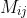to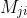is defined by: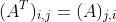﻿
The element in the i row j column is moved to j row i column.
For simplicity, you can imagine that ﻿the determinant is flipped along the principal diagonal line (which is the top-left to bottom-right one).
Example:Even if it isn't square matrix it still works: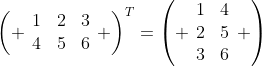In HKDSE, transpose is almost useless so I won't discuss its main properties, except one: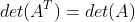It may help when we can't solve a determinants, flipping it gives more clues. Take the HW as example:
Example: Factorize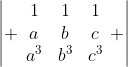, hence evaluate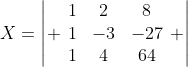.
Now notice that when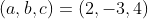, the original one is the transpose of X.
Therefore,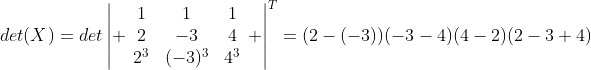(the factorization has been demonstrated before) which gives the answer -210.

Note that transpose has given the symmetricities between rows and columns.

Uniqueness of degree in determinants

Sometimes the factorization does not give a unique degree in the polynomial expression which may cause difficulties in factorization.
Recall that determinants is the sum of product of elements from distinct rows and columns. Therefore it each row or column has unique degree, the factorization result has a unique degree.
For example,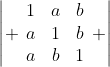In such case you can treat 1 as a variable in order to equate the degree. The determinant becomes:Note that this determinant is NOT cyclic because each row are not in cyclic relationship.
Cyclic group of (a,b,c): (a,b,c) -> (b,c,a) -> (c,a,b) -> (a,b,c)
Cyclic group of (b,a,c): (b,a,c) -> (a,c,b) -> (c,b,a) -> (b,a,c)
They are two different group, and in this example, (c,a,b) can't reach (a,c,b) by cyclic relationship.
However we see that (a,b) is symmetrical becauseTherefore if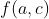is a factor of the det.,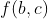is also a factor of the det.
Notice that when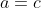,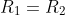,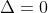(the value of determinant = 0), so (c-a) is a factor of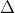. As we have proven, (c-b) is also a factor of.
Though it's not cyclic, each row still contain a,b,c, which tell us (a+b+c) is a factor of it (this is an usual and important practice in factorizing determinants).
Equating degree,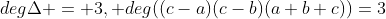, so we have factorized out all factor of, we now assume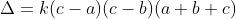, where k is a constant. By equating the coefficient, we get k = 1, and putting c = 1, we have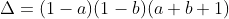Case for x=y=z

When two equal variables "kill" the determinant, we say (a-b) is a factor. How to determine the factor when the condition "x=y=z" kill the determinant?

If x=y=z is the condition to make determinant zero, then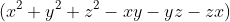is the factor of the determinant.

Prove: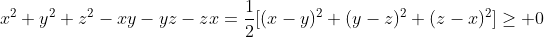. It's zero only if x=y=z, and therefore by factor theorem it's a factor of the determinants.

This is a simplified version of AM-GM inequalities or Rearrangement Inequalities, HKALE players should be familiar with it.

Example:
Factorize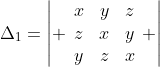, hence show that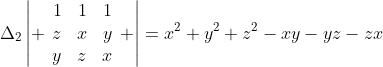Step 0: Notice that the determinant is cyclic.
Step 1: Notice that the determinant vanishes when x=y=z, sois a factor of the determinant.
Step 2: Equating degree, the degree of determinant is 3 while the current factor is 2, that remains one more factor with degree 1, then that must be (a+b+c).
Step 3: Equating coefficient,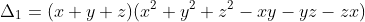Step 4: The second part of the question: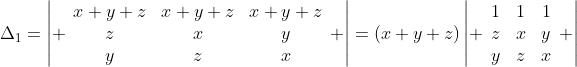Therefore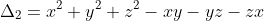.

For ugly result which can't be factorized

In this case we can't do much other than directly expansion.
Example: express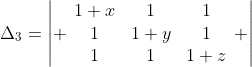in polynomial.
Notice that
1) This is symmetrical (as we have proven)
2) Each term of the polynomal does not contain a term with a square or a cube of a certain variable like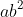.
Then we can conclude that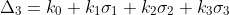, where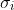are elemental functions for 3 variables.
By equating the coefficient, we have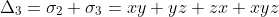.

Example: express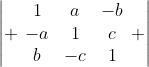in polynomial.
Notice that: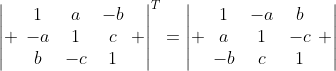, therefore the sign of variable does change the value of determinant. Since the degree is under 3, we deduce that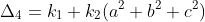where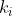are constants. By equating the coefficient, we have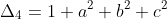.

Exercise:
1)Show that for distinct a,b,c,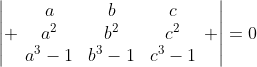, then abc=1.
2)Factorize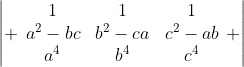given that the product is four linear and one quadratic factors. [Sheffield past paper]
3)Factorize.
4)Show that (a,c) is symmetrical for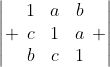, hence express it in polynomial.
5)Show that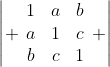is symmetrical, hence express it in polynomial.
6)Why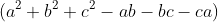is not a factor of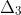?

Extra question:
For a square matrixwhereandare constants. The rest of the entries are 1. Show by induction, or otherwise,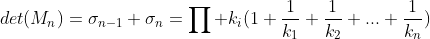.  (AL level; 6~7M)